Courses

# RD Sharma Solutions -Ex-20.3, Mensuration, Class 6, Maths Class 6 Notes | EduRev

## RD Sharma Solutions for Class 6 Mathematics

Created by: Abhishek Kapoor

## Class 6 : RD Sharma Solutions -Ex-20.3, Mensuration, Class 6, Maths Class 6 Notes | EduRev

The document RD Sharma Solutions -Ex-20.3, Mensuration, Class 6, Maths Class 6 Notes | EduRev is a part of the Class 6 Course RD Sharma Solutions for Class 6 Mathematics.
All you need of Class 6 at this link: Class 6

Question 1. The following figures are drawn on a squared paper. Count the number of squares enclosed by each figure and find its area, taking the area of each square as 1 cm2.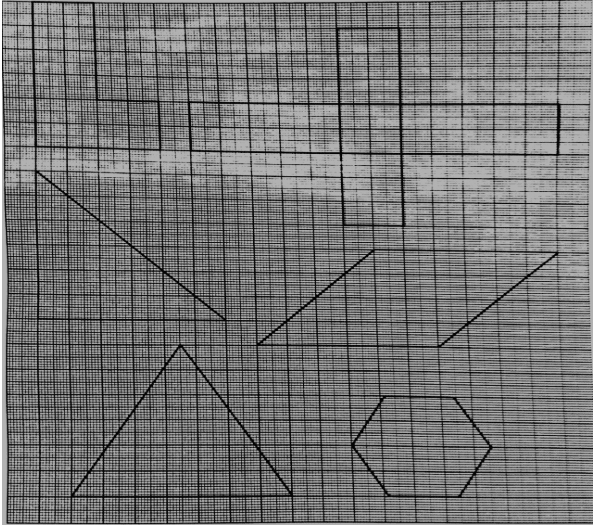Solution:

(i) There are 16 complete squares in the given shape.

Since, Area of one square = 1 cm2

Therefore, Area of this shape = 16 × 1 = 16 cm2

(ii) There are 36 complete squares in the given shape.

Since, Area of one square = 1 cm2

Therefore, Area of 36 squares = 36 × 1 = 36 cm2

(iii) There are 15 complete and 6 half squares in the given shape.

Since, Area of one square = 1 cm2

Therefore, Area of this shape = (15 + 6 × 12) = 18 cm2

(iv) There are 20 complete and 8 half squares in the given shape.

Since, Area of one square = 1 cm2

Therefore, Area of this shape = (20 + 8 × 12) = 24 cm2

(v) There are 13 complete squares, 8 more than half squares and 7 less than half squares in the given shape.

Area of one square = 1 cm2

Area of this shape = (13 + 8 × 1) = 21 cm2

(vi) There are 8 complete squares, 6 more than half squares and 4 less than half squares in the given shape.

Area of one square = 1 cm2

Area of this shape = (8 + 6 × 1) = 14 cm2

Question 2. On a squared paper, draw (i) a rectangle, (ii) a triangle, (iii) any irregular closed figure, Find approximate area of each by counting the number of squares complete, more than half and exactly half.

Solution: (i) A rectangle: This contains 18 complete squares.

If we assume that the area of one complete square is 1 cm2,

Then the area o this rectangle will be 18 cm2.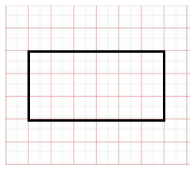(ii) A triangle: This triangle contains 4 complete squares, 6 more than half squares and 6 less than half squares.

If we assume that the area of one complete square is 1 cm2,

Then the area of this shape = (4 + 6 x 1) = 10 cm2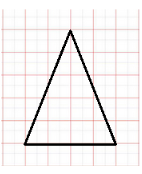(iii) Any irregular figure: This figure consists of 10 complete squares, 1 exactly half square, 7 more than half squares and 6 less than half squares.

If we assume that the area of one complete square is 1 cm2,

Then the area of this shape = (10 + 1 x12 + 7 x 1) = 17.5 cm2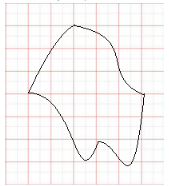Question 3. Draw any circle on the graph paper, Count the squares and use them to estimate the area the area of the circular region.

Solution: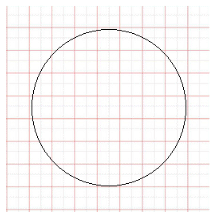This circle on the squared paper consists of 21 complete squares, 15 more than half squares and 8 less than half squares.

Let us assume that the area of 1 square is 1 cm2.

If we neglect the less than half squares while approximating more than half square as equal to a complete square, we get:

Area of this shape = (21 + 15) = 36 cm2

Question 4. Using tracing paper and centimeter graph paper to compare the areas of the following pairs of figures: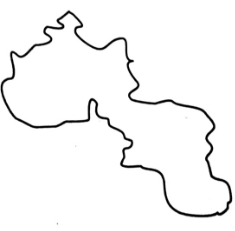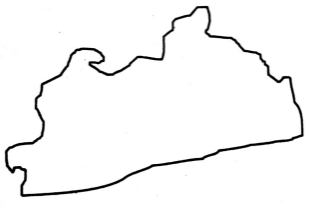Solution: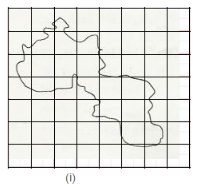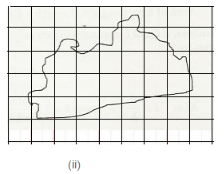Using tracing paper, we traced both the figures on a graph paper.

This figure contains 4 complete squares, 9 more than half squares and 9 less than half squares. Let us assume that the area of one square is 1 cm2

If we neglect the less than half squares and consider the area of more than half squares as equal to area of complete square, we get:

Area of this shape = (4 + 9) = 13 cm2

This figure contains 8 complete squares, 11 more than half squares and 10 less than half squares.

Let us assume that the area of one square is 1 cm2.

If we neglect the less than half squares and consider the area of more than half squares as equal to area of complete square, we get:

Area of this shape = (8 + 11) = 19 cm2

On comparing the areas of these two shapes, we get that the area of Fig. (ii) is more than that of Fig. (i).

103 docs

,

,

,

,

,

,

,

,

,

,

,

,

,

,

,

,

,

,

,

,

,

,

,

,

,

,

,

,

,

,

;